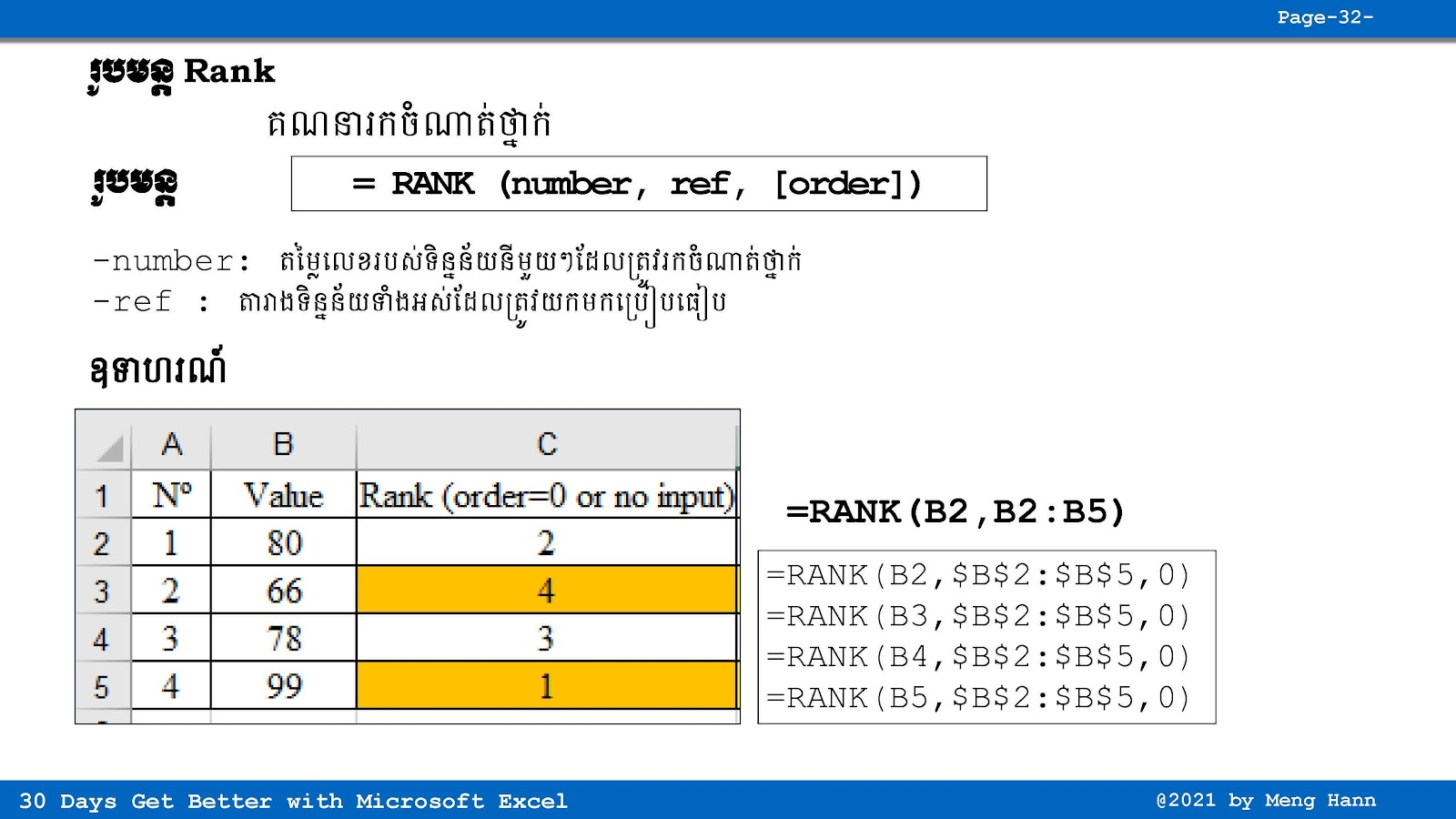# 27. How to use RANK formula in Excel speak Khmer

27. How to use RANK formula in Excel speak Khmer
If you want to find number 1,2,3,... then Rank could help you but it comes with some tricks if you don't understand the concept. Please refer to the video for more information. RANK Formula
=RANK(Number,Ref,[order])
=Average(start_cell:end_cell)
◾ Number: Required, total or average value to find rank
◾ Ref: Required,set of range or cells to compare with number.
◾ [order]: optional